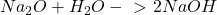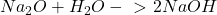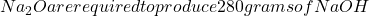## Consider the following equation:How many grams of [tex]Na_{2}O are required

Question

Consider the following equation:How many grams of